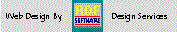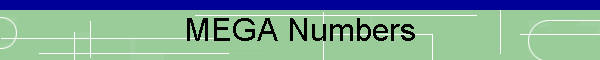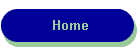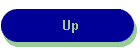RDF-Home

LUGIE
Linux Users Group

Zenbot CNC
User's Group

The chart below shows the frequency of MEGA numbers selected from the most recent Pick-6 Super Lottery Drawings: Game #3104  thru 3030  Updated 01/05/17  For a number analysis of all numbers {including the mega numbers} see: last50+

Last Week's Mega Number Results:

 Game 3104 6 12/31/16 Game 3103 6 12/28/16

Mega  Number Frequency for the California State Lottery

Num=  1 Count=   3    Num= 15 Count=   5

Num=  2 Count=   4    Num= 16 Count=   3

Num=  3 Count=   3    Num= 17 Count=   6

Num=  4 Count=   1    Num= 18 Count=   4

Num=  5 Count=   4    Num= 19 Count=   3

Num=  6 Count=   4    Num= 20 Count=   0

Num=  7 Count=   0    Num= 21 Count=   1

Num=  8 Count=   3    Num= 22 Count=   1

Num=  9 Count=   0    Num= 23 Count=   3

Num= 10 Count=   5    Num= 24 Count=   7

Num= 11 Count=   2    Num= 25 Count=   2

Num= 12 Count=   5    Num= 26 Count=   1

Num= 13 Count=   1    Num= 27 Count=   4

Num= 14 Count=   0

Valid Numbers in this series= 75 from 75 most recent Games

Frequency Range:  Max=7 Min=0

Most Frequent Numbers:

Number   24 Frequency=   7

Target Frequency:   4

Number    2 Frequency=   4

Number    5 Frequency=   4

Number    6 Frequency=   4

Number   18 Frequency=   4

Number   27 Frequency=   4

Least Frequent Numbers:

Number    7 Frequency=   0

Number    9 Frequency=   0

Number   14 Frequency=   0

Number   20 Frequency=   0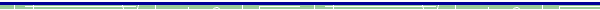dBase, Delphi  and C++ Builder  are trademarks of Borland International, Inc. All other products mentioned are registered trademarks or trademarks of their respective companies. Questions or problems regarding this web site should be directed to RDF@RDFoerster.com. Copyright © 1997-2007 RDFoerster Software. All rights reserved. Last modified: Thursday January 05, 2017